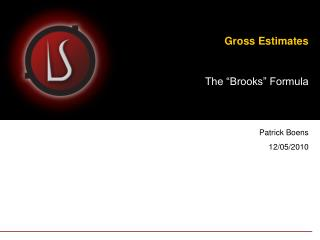DownloadDownload PresentationGross Estimates

# Gross Estimates

Télécharger la présentation## Gross Estimates

- - - - - - - - - - - - - - - - - - - - - - - - - - - E N D - - - - - - - - - - - - - - - - - - - - - - - - - - -
##### Presentation Transcript

1. Gross Estimates The “Brooks” Formula Patrick Boens 12/05/2010

2. Agenda • Background • Context & Objectives • Formula • Base • Updated • Developers’ focus • Supervision • Contingency • Deployment

3. Background • Frederick Brooks is a Software Engineer and Computer Scientist, best-known for managing the development of IBM 0S/360. • Author of the famous “The Mythical Man-Month”, a book about Software Engineering and Project Management. • He had mistakenly added more workers to a project falling behind schedule, considering the equivalence of “resources” and “time” (Man-Month). • In his book, he presents a simple formula for software estimates, a formula he considered to be invalid in subsequent editions. • Still, this formula is a very good basis for early estimates.

4. Context & Objectives • Early stages of a project (inception, initiation) • Gross != precise  it gives an idea, a rough estimate (no equivalence with COCOMO II for example)

5. Base Formula • Effort = 1/3 specs + 1/6 code + 1/4 ut + 1/4 it … where • specs = requirements + analysis • code = build • ut = unit tests • it = integration testing

6. Updated Formula • Some refinements are needed in order to cope with “Prince 2” sort of slicing: • Specs = Requirements + analyses (BA, FA, TA) = 1/3 = 4/12 • Code = Code + Unit tests = 1/6 + 1/4 = 5/12 • Tests = Integration Testing + Functional Testing* = 1/4 = 3/12 *UAT is not included (not a concern of IT)

7. Updated Formula • Supervision effort (PM and Team Leader work)  not included • Contingency  not included • Deployment effort  not included • The formula becomes: • Specs = Requirements + analyses = 1/3 = 4/12 • Code = Code + Unit tests = 1/6 + 1/4 = 5/12 • Tests = Integration Testing + Functional Testing = 1/4 = 3/12 • + supervision + contingency + deployment effort

8. Updated formula: Developers’ focus • Developers focus on: • Specs = Requirements + analyses = 1/3 = 4/12 • Code = Code + Unit tests = 1/6 + 1/4 = 5/12 • Tests = Integration Testing + Functional Testing = 1/4 = 3/12 • + supervision + contingency + deployment effort

9. Updated formula: Supervision • Supervision is often seen as a percentage of the overall project (± 15%*) • Example: Specs + Code + Tests = 100 mdays  Supervision = 15 mdays • Total effort = 100 mdays + 15 mdays = 115 mdays * Although acceptable, a more precise formula exists to calculate the Supervision effort. we’ll see this in a minute

10. Updated formula: Contingency • Contingency is a way to foresee what’s impredictable. Statistically, 17% contingency is used. This percentage must be adapted to the context of your project, based on the degree of uncertainty. • Example: Specs + Code + Tests + Supervision = 115 mdays  Contingency = 20 mdays • Total effort = 100 mdays + 15 mdays + 20 mdays = 135 mdays

11. Updated formula: an example • Imagine a developer’s evaluation of 50 mdays, and now calculate the overall effort  1/12 = 10 mdays • specs = 4/12 = 40 mdays • code = 5/12 = 50 mdays • tests* = 3/12 = 30 mdays • Effort = 40 mdays + 50 mdays + 30 mdays = 120 mdays • Supervision = 120 mdays x 15/100 = 18 mdays • Contingency = 138 mdays x 17/100 = 23 mdays • Deployment = x mdays (say, 5)  Total effort = 161 mdays + 5 mdays = 166 mdays * UAT not included

12. Updated formula: the bottom line • You started with 50 mdays and you ended up with 166 mdays! • This sounds funny: an experienced Project Manager said that he always multiplied the developers’ evaluations by PI  50 mdays x 3.141592 = 157 mdays! ... which is close enough to the end result (it remains a gross figure!) * UAT not included

13. Supervision Effort : ≠ 15% • Time and team size must be balanced (theoretical view). A project of 6 months should be worked out with 6 people (team size and duration are equilibrated = square root). Always favor “more time” instead of “more people” (get back to Brooks’ findings). • A PM is needed for the whole duration of the project. If a month work is 20 days of work, you need the PM during 6 x 20 days, x% of his time. • The direct span of control is about 6 people*  for a project of 3 months, you need the PM half of his time; for a 6 months project, you need him full-time. • Getting back to our example of 50 mdays of developers’ estimates  we have calculated an effort of 120 mdays  120 / 20 = 6  SQRT(6) = 2.45  team of 2 people and timeline of 3 months ( 2 x 20 x 3 = 120 mdays) (ideal view)  PM is needed 33% of his time (2 persons for a possible span of 6) during 3 months  Supervision effort = 3 x 20 x 1/3 = 20 mdays (instead of 18 mdays with 15%). • Contingency of 17%  (120 + 20 ) mdays x 17/100 = 24 mdays • Deployment (5 mdays)  Total effort = 120 + 20 + 24 + 5 = 169 mdays  remember, you started from 50! * Software industry